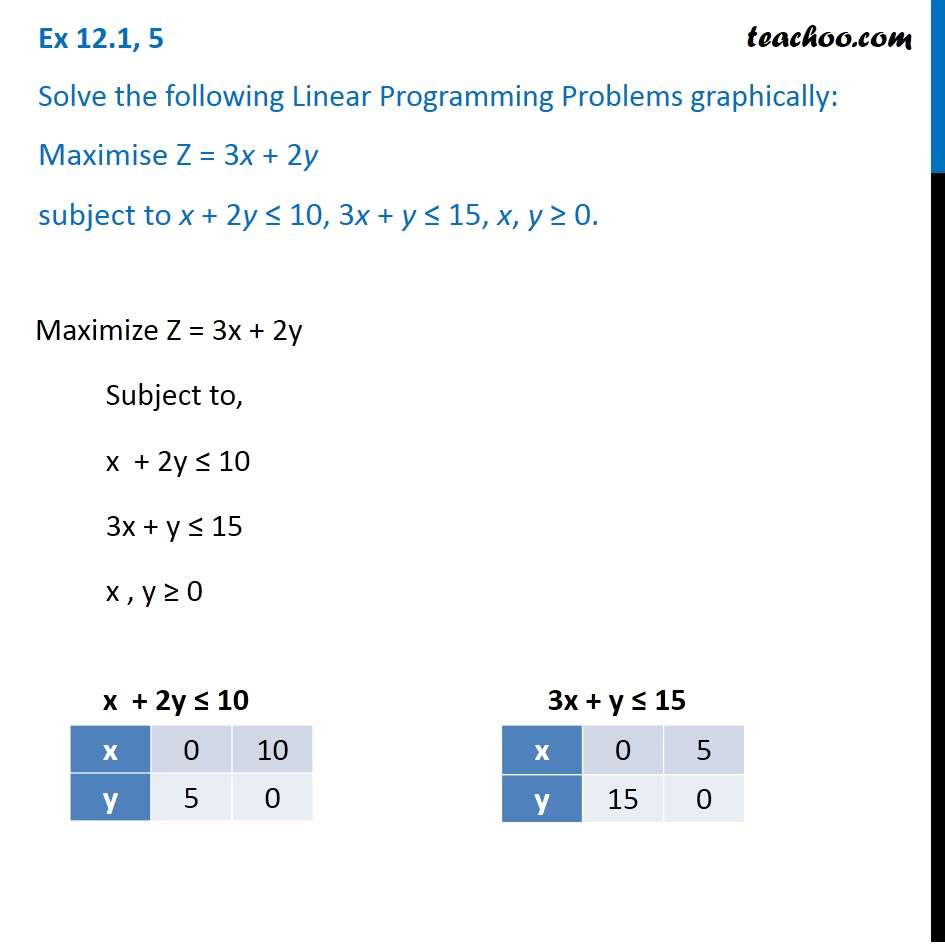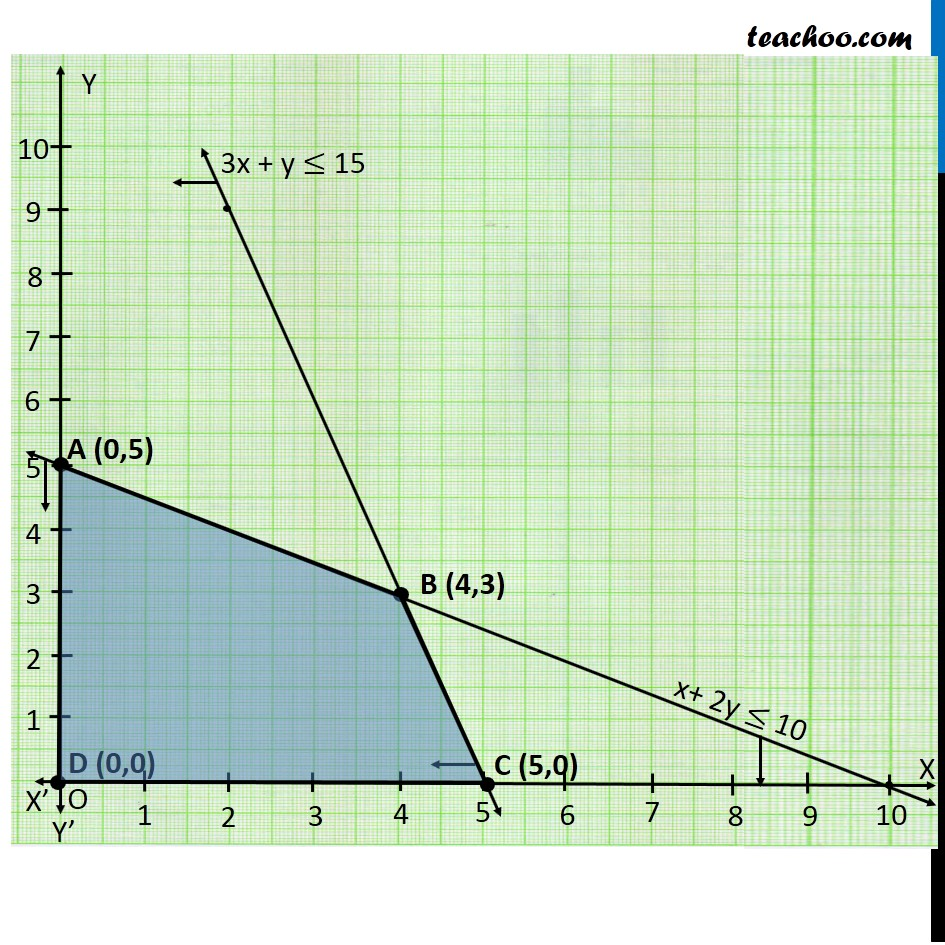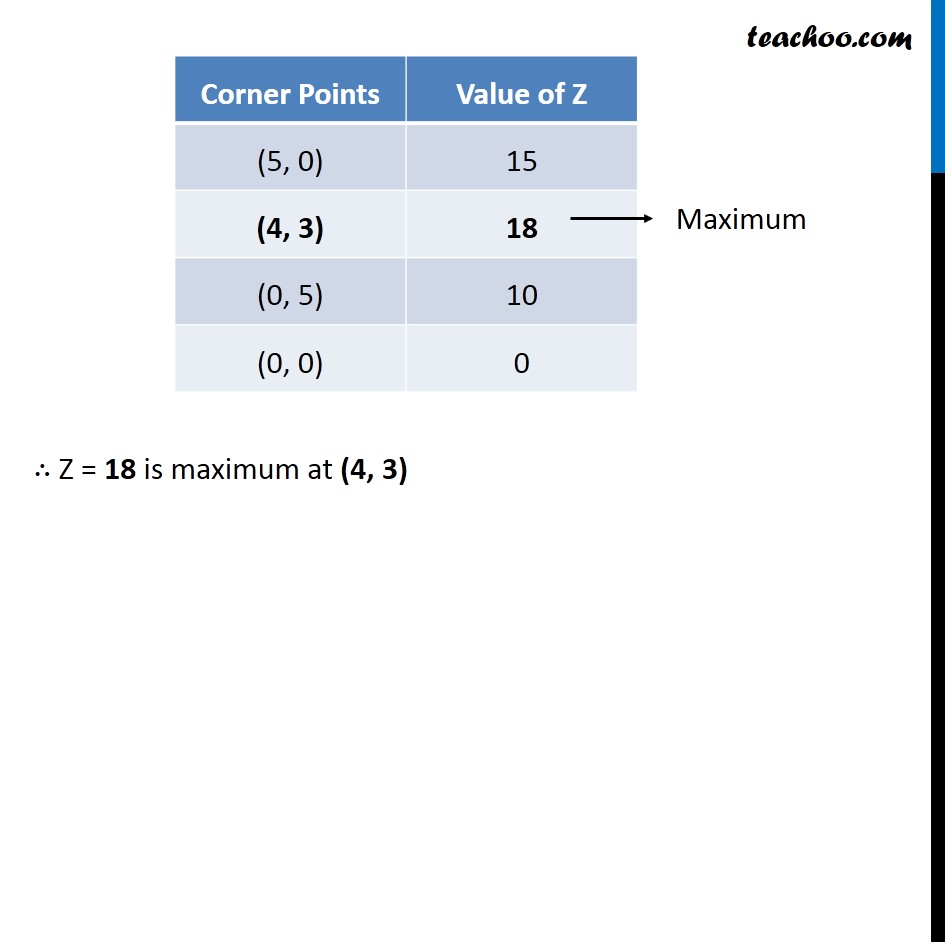Ex 12.1

Chapter 12 Class 12 Linear Programming
Serial order wiseLearn in your speed, with individual attention - Teachoo Maths 1-on-1 Class

### Transcript

Ex 12.1, 5 Solve the following Linear Programming Problems graphically: Maximise Z = 3x + 2y subject to x + 2y ≤ 10, 3x + y ≤ 15, x, y ≥ 0. Maximize Z = 3x + 2y Subject to, x + 2y ≤ 10 3x + y ≤ 15 x , y ≥ 0 ∴ Z = 18 is maximum at (4, 3)